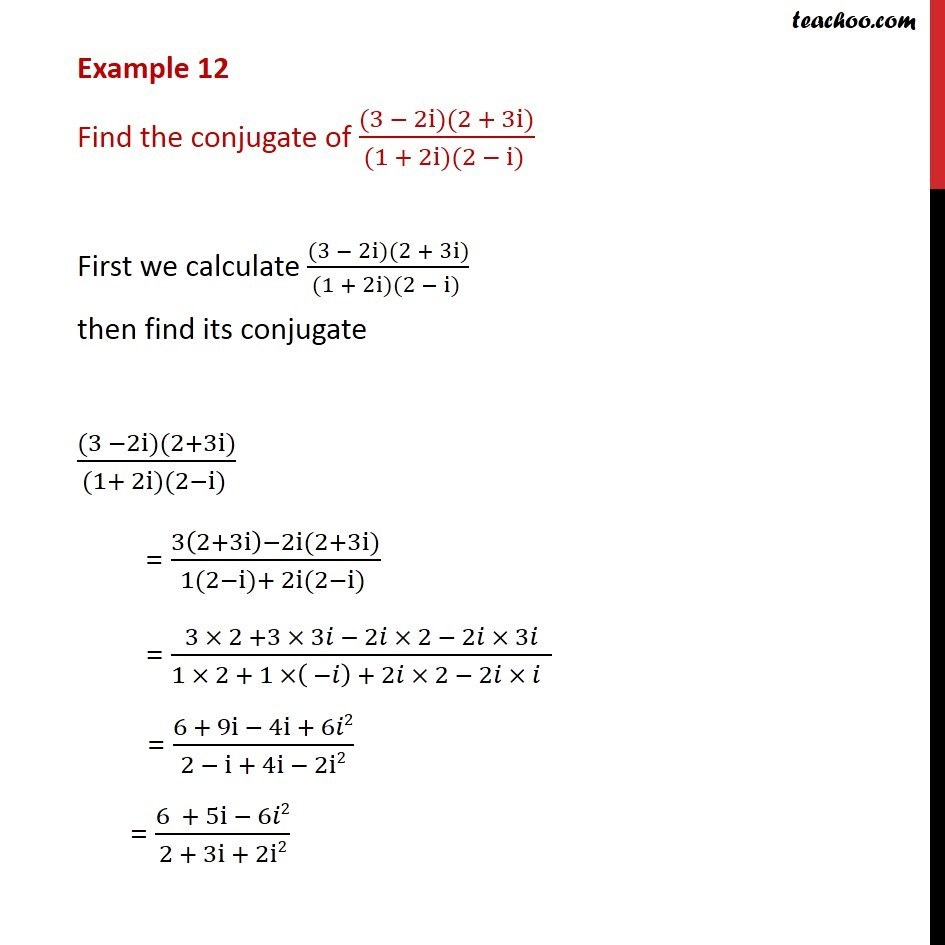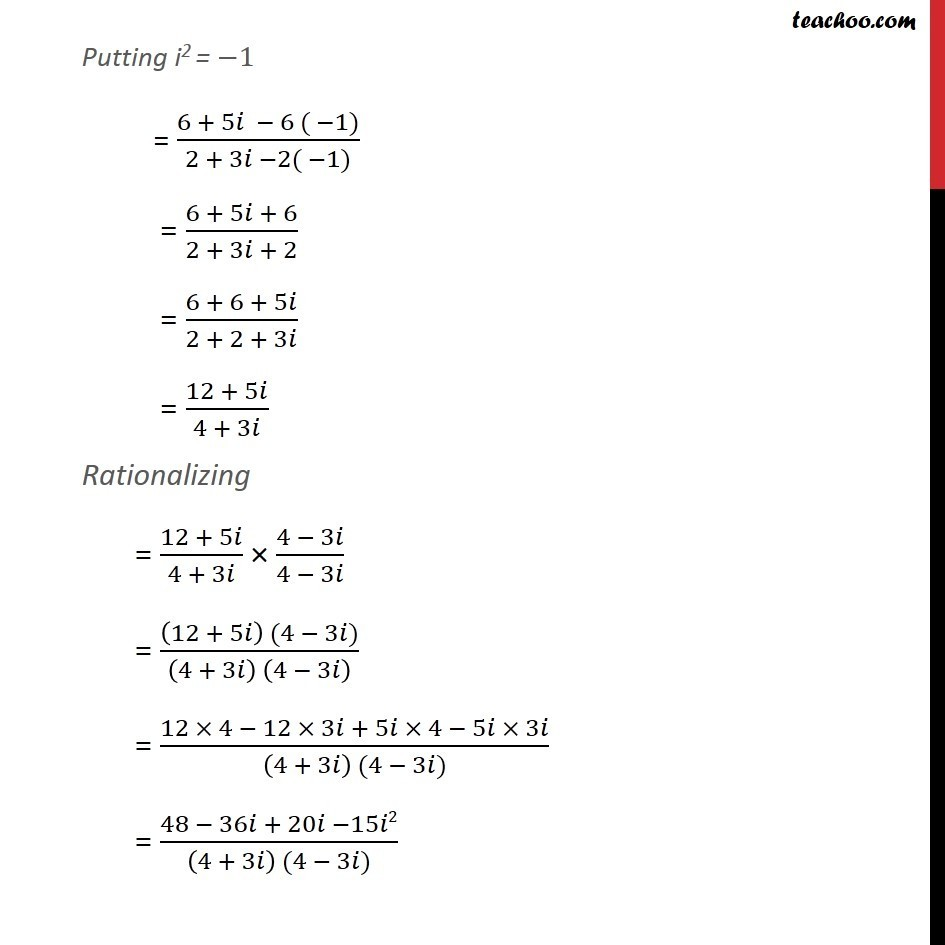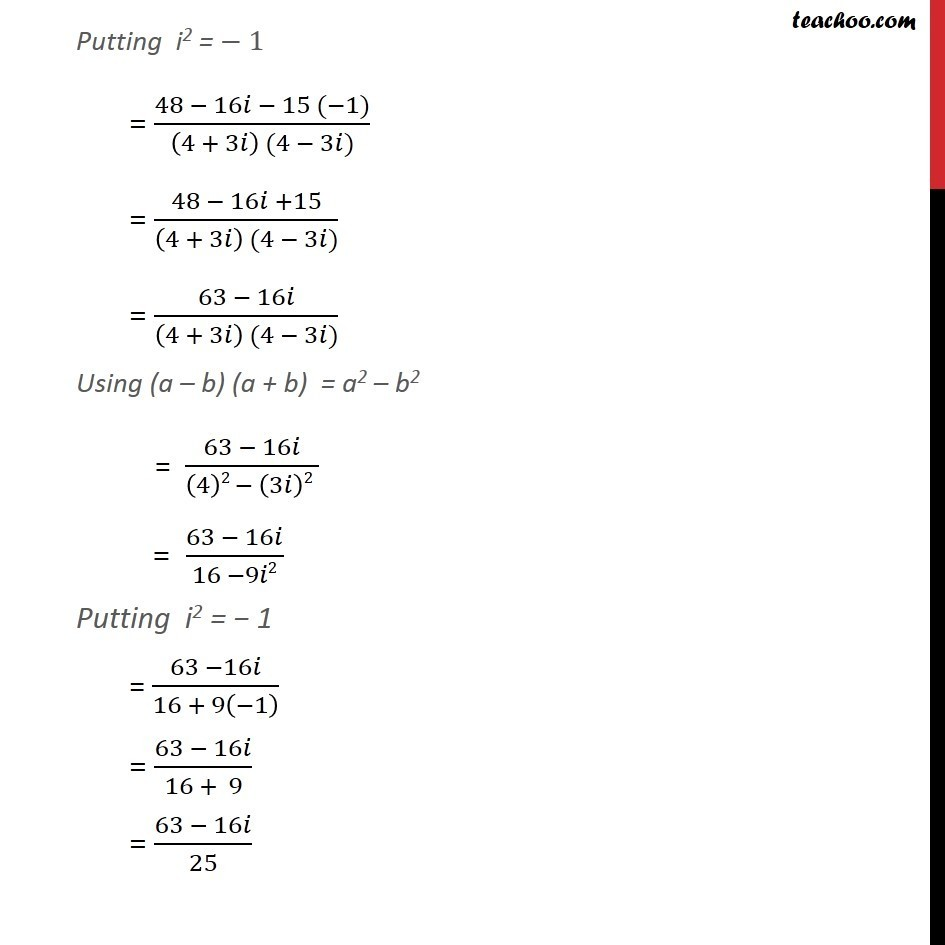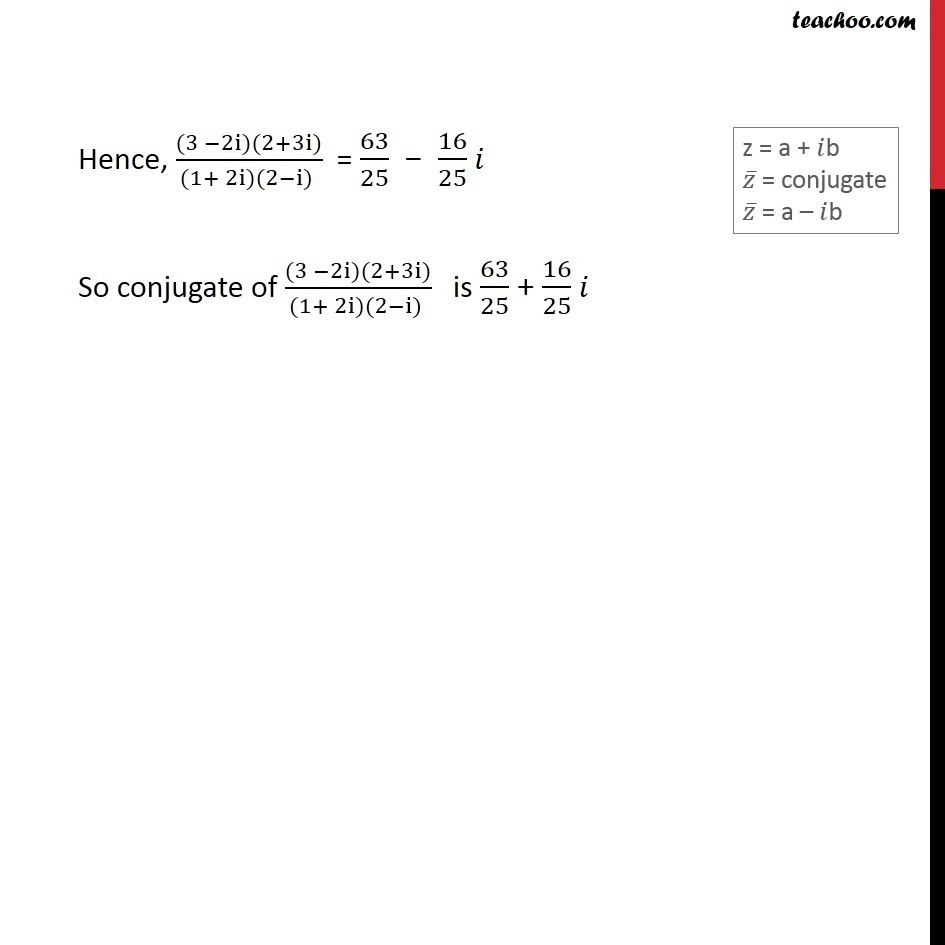Examples

Chapter 4 Class 11 Complex Numbers
Serial order wiseLearn in your speed, with individual attention - Teachoo Maths 1-on-1 Class

### Transcript

Example 7 Find the conjugate of ((3 − 2i)(2 + 3i))/((1 + 2i)(2 − i) ) First we calculate ((3 − 2i)(2 + 3i))/((1 + 2i)(2 − i) ) then find its conjugate ((3 −2i)(2+3i))/((1+ 2i)(2−i) ) = (3(2+3i)−2i(2+3i))/(1(2−i)+ 2i(2−i) ) = (3 × 2 +3 × 3𝑖 − 2𝑖 × 2 − 2𝑖 × 3𝑖)/(1 × 2 + 1 ×( −𝑖) + 2𝑖 × 2 − 2𝑖 × 𝑖 ) = (6 + 9i − 4i + 6𝑖2)/(2 − i + 4i − 2i2) = (6 + 5i − 6𝑖2)/(2 + 3i + 2i2) Putting i2 = −1 = (6 + 5𝑖 − 6 ( −1))/(2 + 3𝑖 −2( −1)) = (6 + 5𝑖 + 6)/(2 + 3𝑖 + 2) = (6 + 6 + 5𝑖)/(2 + 2 + 3𝑖) = (12 + 5𝑖)/(4 + 3𝑖) Rationalizing = (12 + 5𝑖)/(4 + 3𝑖) × (4 − 3𝑖)/(4 − 3𝑖) = ((12 + 5𝑖) (4 − 3𝑖))/((4 + 3𝑖) (4 − 3𝑖) ) = (12 × 4 − 12 × 3𝑖 + 5𝑖 × 4 − 5𝑖 × 3𝑖)/((4 + 3𝑖) (4 − 3𝑖)) = (48 − 36𝑖 + 20𝑖 −15𝑖2)/((4 + 3𝑖) (4 − 3𝑖)) Putting i2 = − 1 = (48 − 16𝑖 − 15 (−1))/((4 + 3𝑖) (4 − 3𝑖)) = (48 − 16𝑖 +15)/((4 + 3𝑖) (4 − 3𝑖)) = (63 − 16𝑖)/((4 + 3𝑖) (4 − 3𝑖)) Using (a – b) (a + b) = a2 – b2 = (63 − 16𝑖)/((4)2 − (3𝑖)2 ) = (63 − 16𝑖)/(16 −9𝑖2) Putting i2 = − 1 = (63 −16𝑖)/(16 + 9(−1) ) = (63 − 16𝑖)/(16 + 9) = (63 − 16𝑖)/25 Hence, ((3 −2i)(2+3i))/((1+ 2i)(2−i) ) = 63/25 − 16/25 𝑖 So conjugate of ((3 −2i)(2+3i))/((1+ 2i)(2−i) ) is 63/25 + 16/25 𝑖